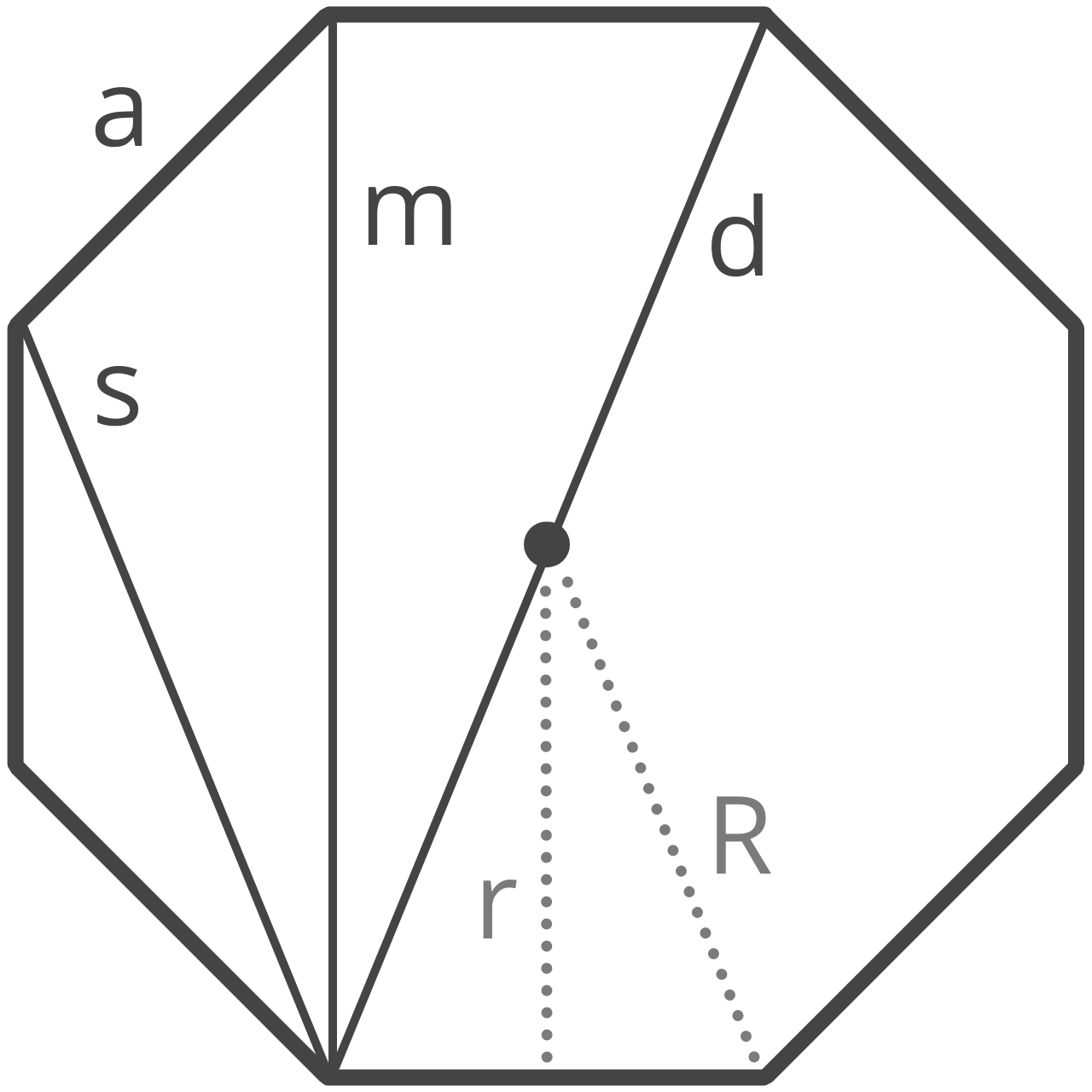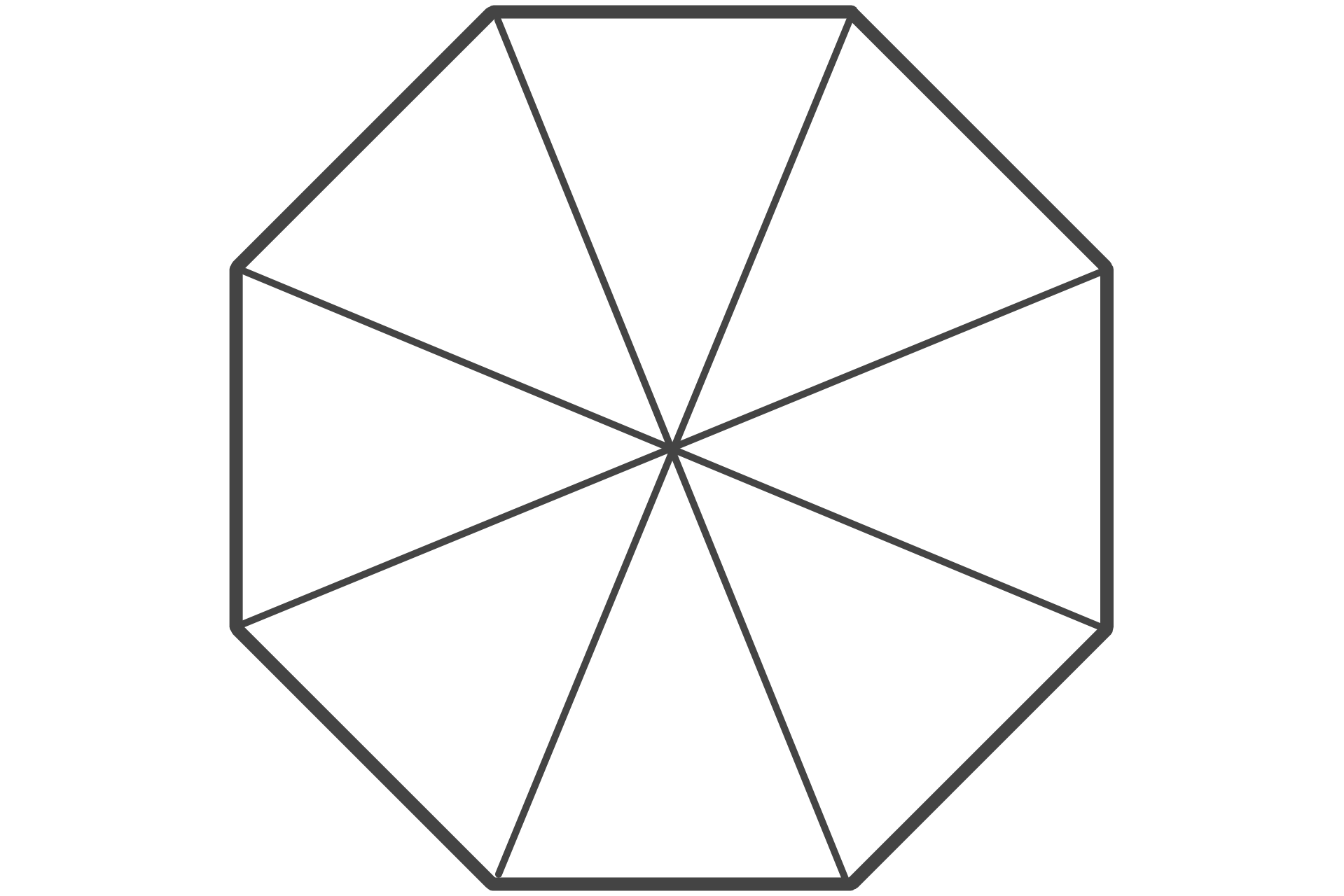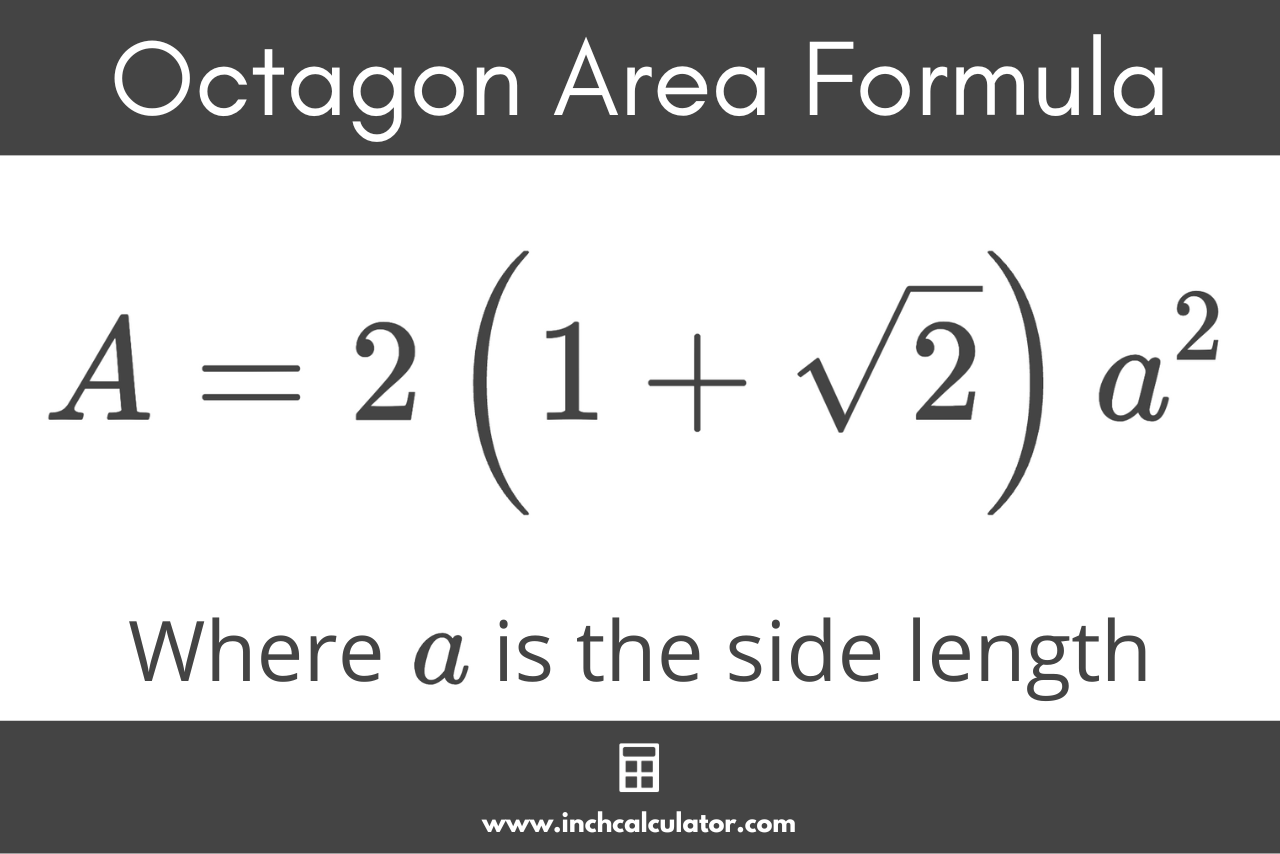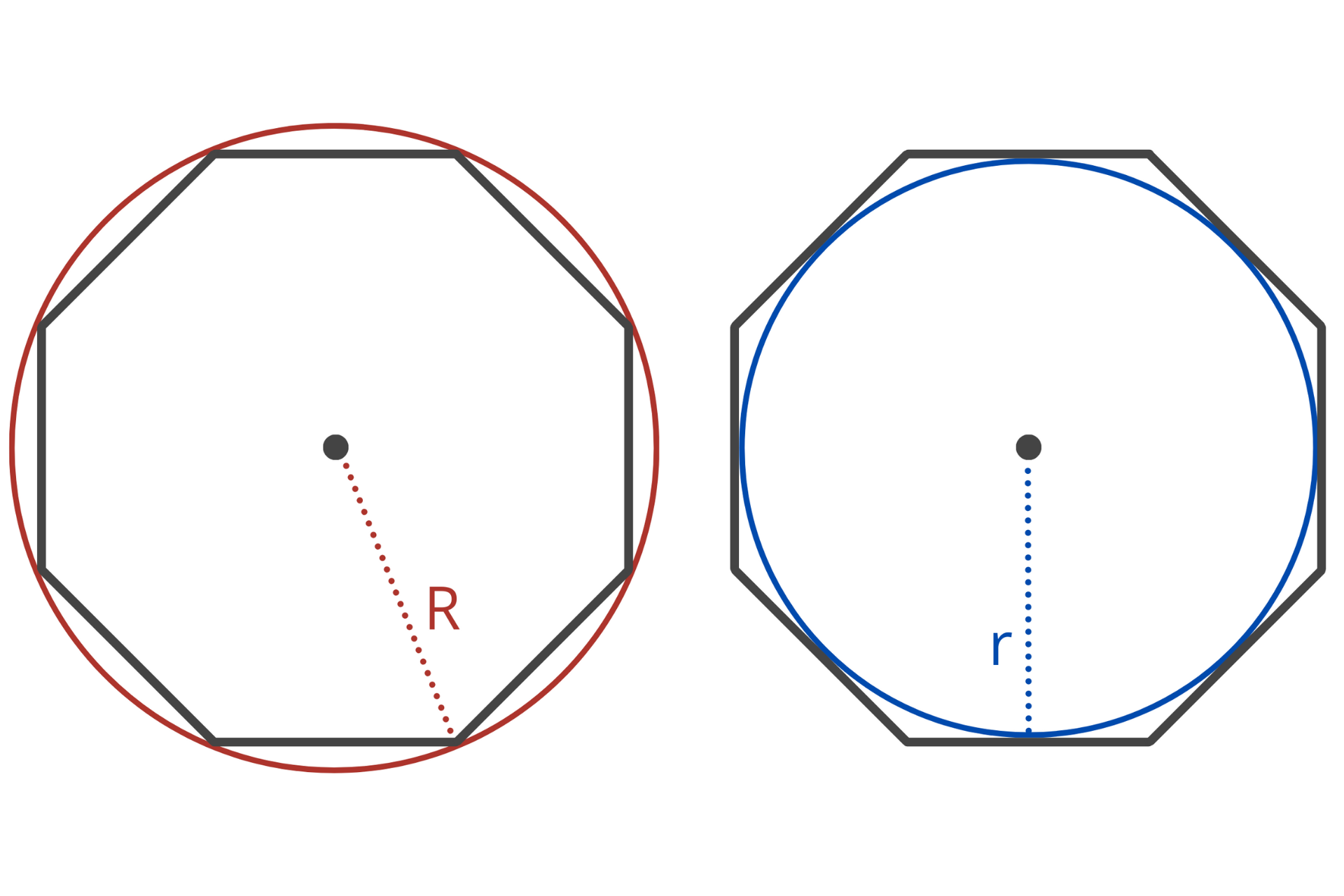# Octagon Calculator

Calculate any property of an octagon using the octagon calculator below.## Solution:

 Side (a): 5 Area (A): 120.71 Perimeter (P): 40 Long Diagonal (d): 13.066 Medium Diagonal (m): 12.071 Short Diagonal (s): 9.239 Circumradius (R): 6.533 Inradius (r): 6.036
Learn how we calculated this below

## How to Calculate the Properties of an Octagon

An octagon is a two-dimensional geometric shape with eight straight sides. The properties of an octagon include the length of the sides a, the area A, the perimeter P, the long diagonal d, the medium diagonal m, the short diagonal s, the radius (circumradius) R, and apothem (inradius) r.

An octagon is a polygon with eight sides and eight angles. When all its sides and angles are equal, it’s referred to as a ‘regular’ octagon. In essence, a regular octagon is symmetric, with all its internal angles measuring 135 degrees, and it can be divided into eight isosceles triangles of equal size.### How to Calculate the Area of an Octagon

To find the area of a regular octagon, you can use the octagon area formula:

A = 2 \left ( 1 + \sqrt{2} \right ) a^{2}

The area A of an octagon is equal to 2 times 1 plus the square root of 2, times the length of the sides a squared. The silver ratio of an octagon is equal to 1 + √2, so the area of an octagon is equal to 2 times the silver ratio times the length of the sides a squared.### How to Calculate the Diagonals of an Octagon

In a regular octagon:

• A short diagonal connects two non-adjacent vertices that have one vertex between them.
• A medium diagonal connects two non-adjacent vertices with two vertices between them.
• A long diagonal connects two non-adjacent vertices with three vertices between them.

You can calculate the length of the diagonals using a few formulas.

#### Short Diagonal

The formula to calculate the short diagonal of a regular octagon is:

s = a \sqrt{2 + \sqrt{2}}

The short diagonal s is equal to the side length a times the square root of 2 plus the square root of 2.

#### Medium Diagonal

The formula to calculate the medium diagonal of a regular octagon is:

m = a \left ( 1 + \sqrt{2} \right )

The medium diagonal m is equal to the side length a times 1 plus the square root of 2. This can also be expressed as the side length a times the silver ratio.

#### Long Diagonal

The formula to calculate the long diagonal of a regular octagon is:

d = a \sqrt{4 + 2 \sqrt{2}}

The long diagonal d is equal to the side length a times the square root of 4 plus 2 times the square root of 2.

Just like a hexagon or triangle, an octagon also has a circumradius and inradius.

The circumradius, or radius, of an octagon is the radius of the circumscribed circle that passes through all the vertices of the octagon.

The inradius, or the apothem, of an octagon is the perpendicular distance from the center of the octagon to one of its sides. In a regular octagon, this is also the height of one of the isosceles triangles when split.You can calculate the circumradius and apothem using a few formulas.